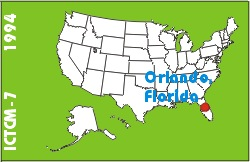# Statistics and Probability Interactive

### Vince DeBlase

The Parkville Center for Mathematics, Science, and Computer Science

### Sharon Wagner

The Parkville Center for Mathematics, Science, and Computer Science

## ABSTRACT

The Parkville Center for Mathematics, Science, and Computer Science opened September, 1994, for ninth grade students. The students will be taking algebra or probability/statistics and functions, geometry, chemistry, and computer science. The computer science class will introduce the student to various software packages such as MicrosoftWorks, Geometry Inventor, Algebra Explorer, Hypercard, and MathCAD. The students will perform the labs in computer science class. The purpose of the labs is to engage the students into becoming active participants in the learning process. The labs provide 'engines' for exploring statistical concepts; i.e. the standard deviation calculator, Chebyshev's viewer, boxplot maker, binomial experiment calculator, correlation calculator, and the regression reporter. Simulations in probability can be done using the die roller, two dice roller, coin tosser, probability of same birthday simulator, and craps game simulator.

The poster session will include a demonstration of the labs. Discussion will focus on authoring software, the authoring process, the technology needed for implementation, and the time involved for development. The presenters will share their classroom experiences using the interactive textbook.

Keyword(s): statistics, probability